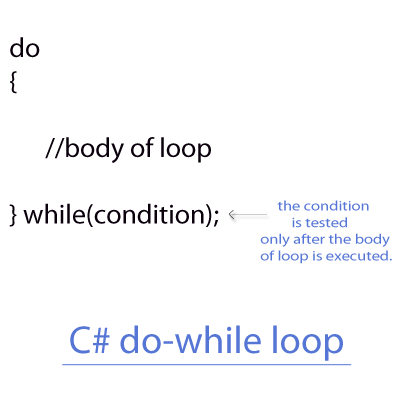< Prev
Next >

# C# Do-While Loop

After discussing the while loop in our previous tutorial, let us discuss another looping structure - do while loop in C#. In do-while loop, a block of statements or the body of loop always comes before the condition of do-while loop is tested.

• Hence, during the first run of do-while loop, the body of do-while loop is always executed, even when the condition of do-while loop is false.

• But from the second execution of do-while loop, the body of loop is only executed when the condition of do-while loop is true.

Note:
• Similar to Java, the C# language also requires a pair of braces { } to hold more than one statement i.e. multiple statements, which are to be executed within a do-while loop.## Example of do-while loop

In the upcoming example, we are going to use do-while loop to print a series of numbers, starting from 0 to 4.

``````//C# Exmaple do-while loop

using System;

class DoWhileEx
{
public static void Main()
{
//Initializing the value of i
int i=0;

do
{
Console.WriteLine("i = " + i);

//Iterating the value of i
i++;
}while(i<5); //Condition of do-while loop

}
}``````

## Output

``````i = 0
i = 1
i = 2
i = 3
i = 4``````

## The do-while loop is executed at least once

The body of the do-while loop is always executed during its first execution, even when the condition of loop is evaluated to be false, take a look at our next example -

``````//C# do-while example

using System;

class DoWhile
{
public static void Main()
{
//Initializing the value of i
int i=100;

do
{
Console.WriteLine("i = " + i);

//Iterating the value of i
i++;

}while(i<10);	//Condition of do-while loop

}
}``````

## Output

``i = 100``

< Prev
Next >
< C# While Loop
C# Break Statement >

Please subscribe to our social media channels for daily updates.